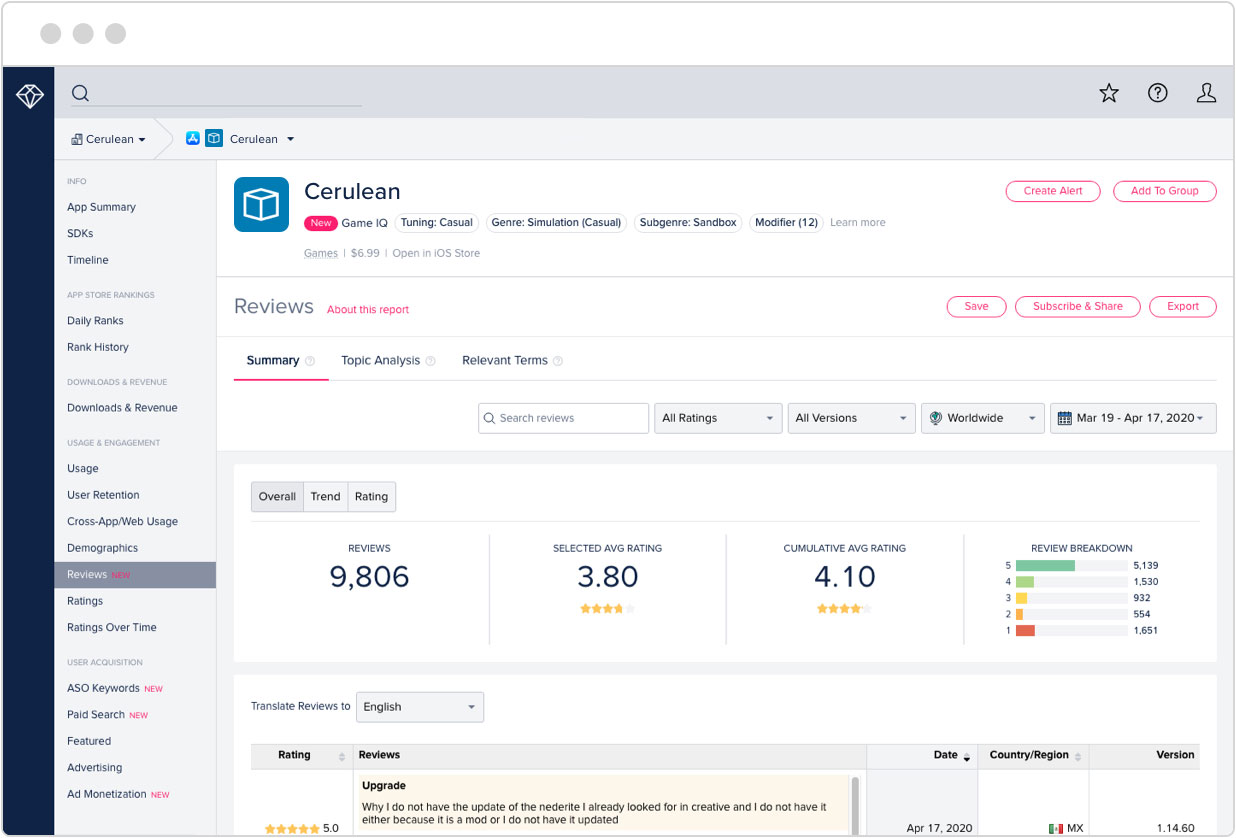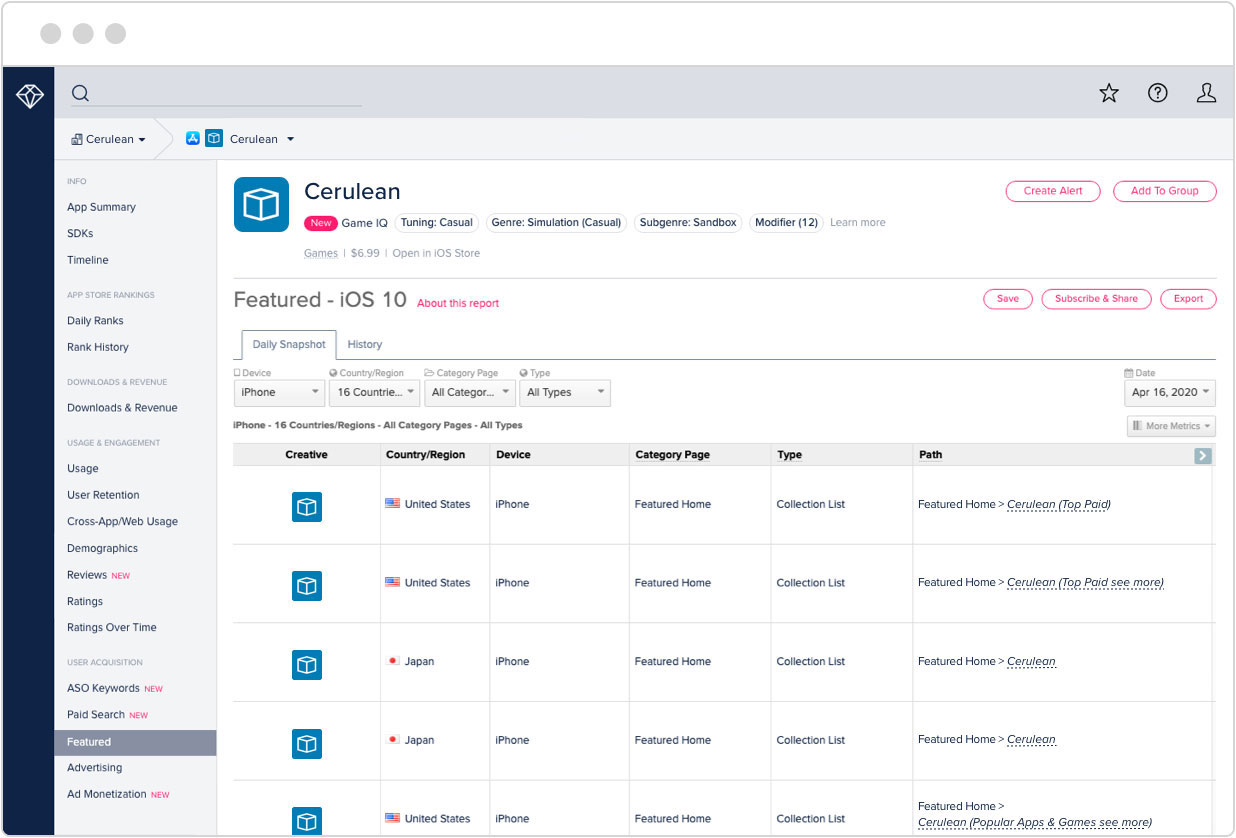Top des applis

Recherchez n'importe quelle app# Geometry PRO

Éditeur: NaNSolvers
Prix: 2.42 USD

### Historique de classement des apps

Consulter l'historique des classements de téléchargement pour Geometry PRO en United States.

L'Historique des classements indique la popularité de Geometry PRO sur Google Play app store et son évolution au fil du temps. Vous pouvez suivre la performance de Geometry PRO pour chaque heure de chaque jour sur différents pays, catégories et appareils.

### Découvrez-en plus après l'inscription gratuite !### Optimisation pour les app stores

Suivez les mots-clés les plus performants pour chaque app référencée, ainsi que les tendances de recherche d'une app au fil du temps pour les mots-clés déterminants.

### Évaluations et notes de l'appli

Obtenez des retours d'utilisateurs importants en parcourant les évaluations et les notes des apps. Recoupez les données par version, par pays et par plage de dates pour découvrir des bugs, suivre les suggestions et améliorer votre app.### Contenus phares

Découvrez où et quand les apps ont été référencées sur les différents app stores par jour, pays, périphérique et catégorie.

### Description de l'appli

Geometry PRO is advanced application for solving geometry problems. There is a complete solution delivered for each issue to satisfy every teacher or student.

The application solves every algebraic problem including those with:
- fractions
- roots
- powers
you can also use parentheses, decimal numbers and Pi number.

This application is able to do calculation on the following figures:
- square
- rectangle
- rhombus
- parallelogram
- triangle
- equilateral triangle
- right triangle
- isosceles triangle
- triangle 30-60-90
- circle
- annulus
- trapezoid
- right trapezoid
- isosceles trapezoid
- Pythagorean theorem
- regular hexagon
- sphere
- cylinder
- cone
- regular tetrahedron
- cube
- square prism
- cuboid
- ellipse
- regular Pentagon
- Kite
- trigonometry
- spherical sector
- spherical cap
- annulus sector

PRO version:
- Square pyramid
- Triangular pyramid
- Triangular prism
- Regular triangular prism
- Thales' theorem
- Truncated cone
- Regular octagon
- Regular dodecagon
- Hexagonal prism
- Hexagonal pyramid
- Pentagonal prism
- Barrel
- law of sines
- law of cosines
- spherical wedge
- spherical lune
- spherical segment
- spherical zone

In addition, the application has the following features:
ANALYTIC GEOMETRY
- Points and lines
- Intersection point
- Distance from point
- Length of the segment
- Parallel and Perpendicular line
- Perpendicular bisector
- Axial symmetry
- Central symmetry
- Translation by a vector
- Angle between lines
- Angle bisector
- Bisector of the angle between two lines
- Value of the angle from three points
- Position of a point relative to a line
- Relative position of two lines
- Relative position of three points
- Relative position of two circles
- Relative position of a circle and a line
- Relative position of a circle and a point
- Translation of a circle by a vector
- Circle reflection over point
- Circle reflection over line
- Circle with radius and two points
- Circle with center and point
- Circle with center and radius
- Circle with three points
VECTORS
- 2D and 3D
- Length of a vector
- Dot product
- Cross product

An advanced validation of data entry allows you to find the errors quickly and corrects them for you immediately.

Geometry PRO will calculate all the parameters of the figure, if you enter the necessary data. The order of data entry depends on you!

- Do you want to calculate a side of the square? No problem. Geometry PRO will do it for you.
- Do you have an angle and a side of the right triangle? Perfect. Other values can be calculated.

None of your geometry tasks will be a problem now with Geometry PRO. This application has very advanced, powerful and easy - to - use interface.

Additionally, it contains all the useful formulas which you will need to solve geometry tasks. But thats not enough! You do not have to figure it out how did you get a result. This application not only gives you the solution, it also shows you all the formulas which had been used. Pythagorean Theorem, sines and cosines are no longer a problem.

### Optimisation pour les app stores

Quels mots les gens saisissent-ils lorsqu'ils cherchent une appli ? Les bons mots-clés peuvent aider une appli à se faire connaître, ce qui augmente les téléchargements et le chiffre d'affaires. data.ai suit des millions de mots-clés afin que vous puissiez augmenter les téléchargements de votre appli et savoir quels mots-clés vos concurrents utilisent.

### Évaluations et notes de l'appli

Découvrez ce que les utilisateurs pensent de Geometry PRO en lisant les évaluations et en consultant la note.

## 0

#### 0 notes

Super

Apr 09, 2022

Works great 👍

May 11, 2022

Unable to use clipboard to copy results or paste parameters

Apr 09, 2022

Works great 👍

May 11, 2022

Great set of maths apps. Shame you do not do a pack of all the pro.

May 09, 2022